深入EVM：EVM工作机制的深层分析（终极版）Getting Deep Into Geth: Why Syncing Ethereum Node Is Slow

Getting Deep Into Ethereum: How Data Is Stored In Ethereum?

5 resources to get started with ethereum

EVM概览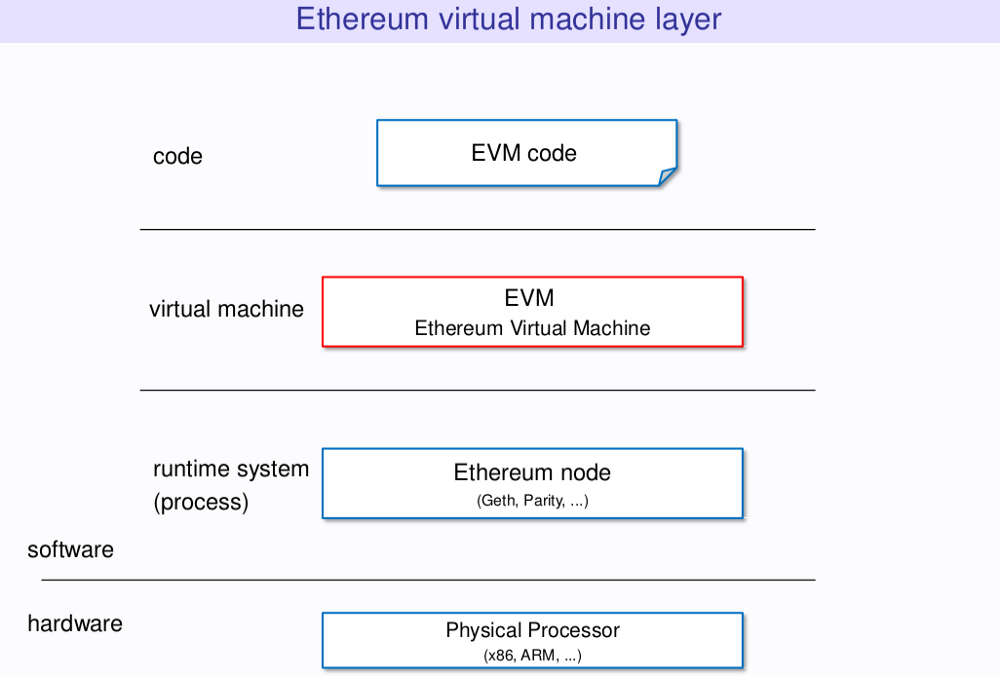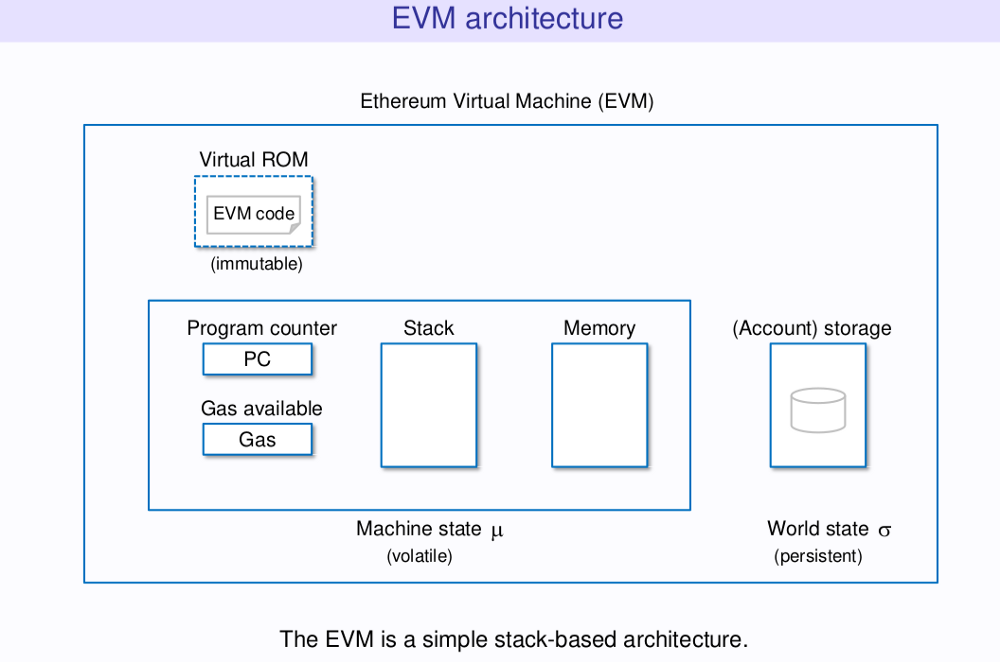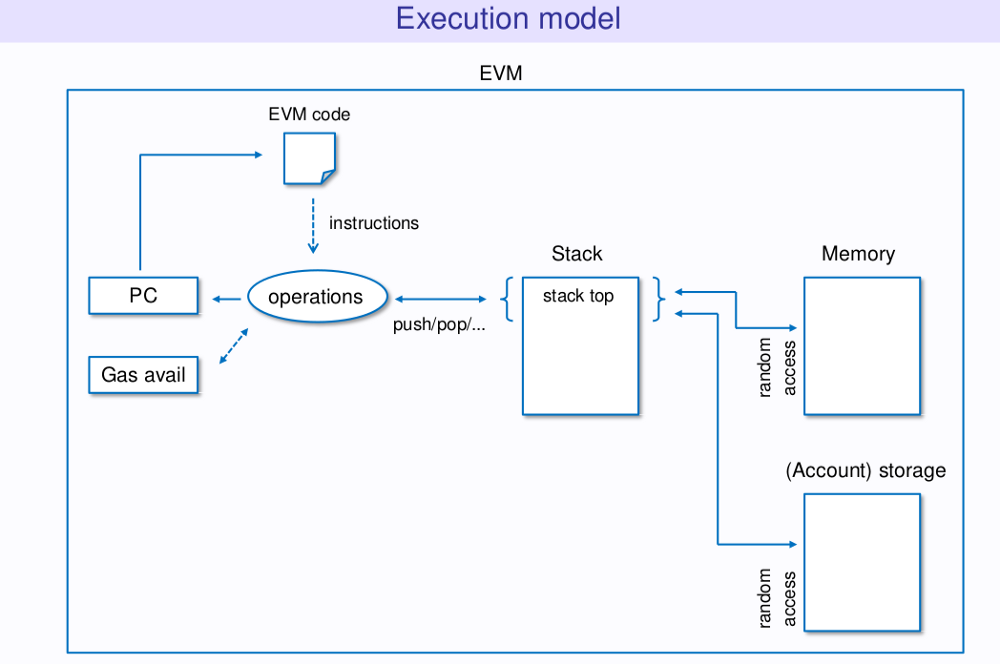以太坊合约（Ethereum Contracts）

基本概念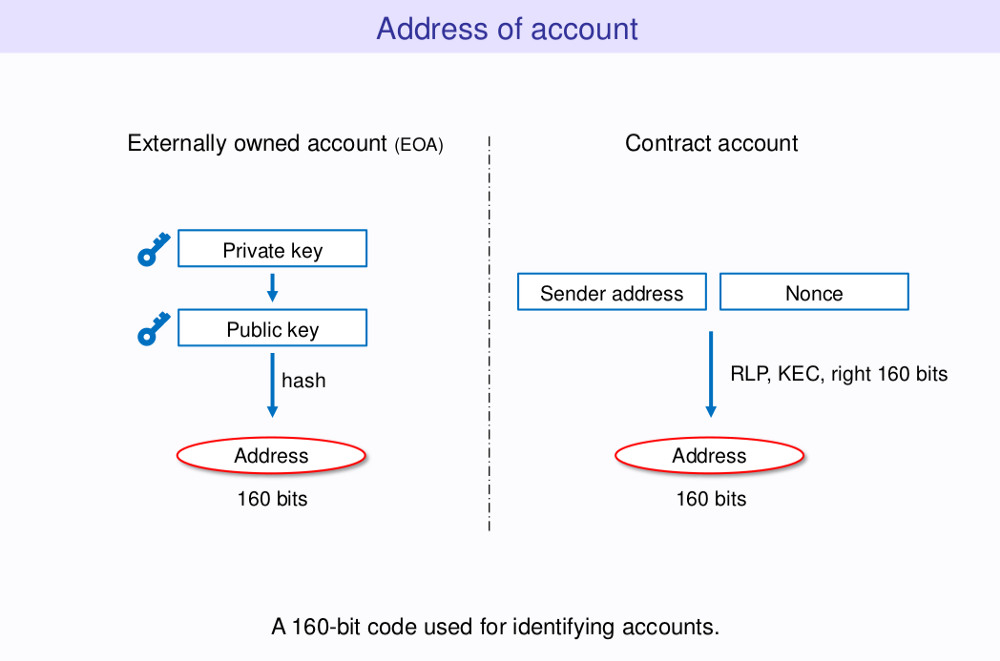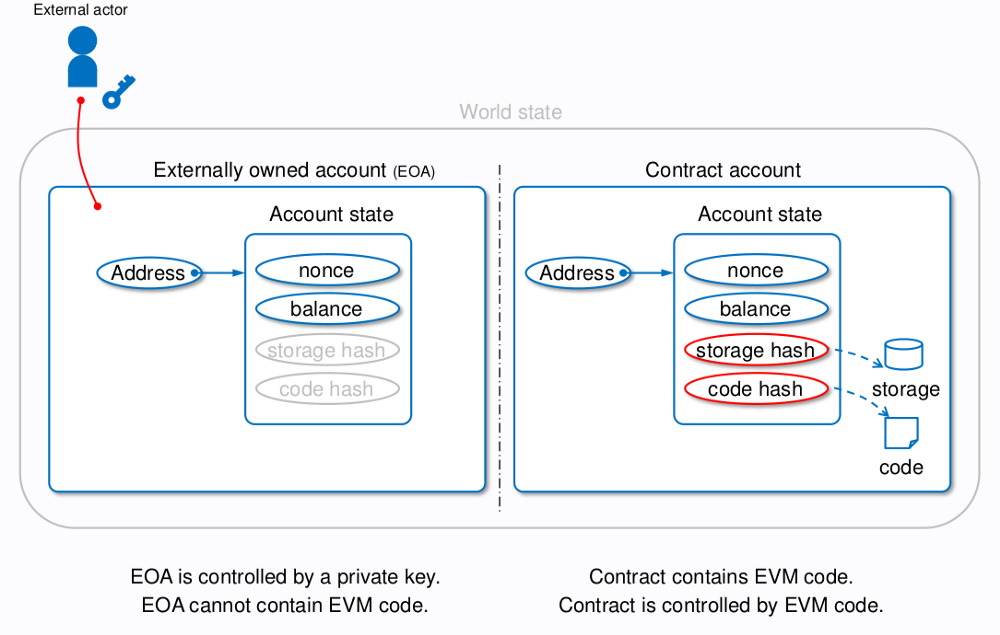创建合约

``````pragma solidity ^0.4.21;
contract MyContract {
function MyContract() public {
emit Log(this);
}
function add(uint256 a, uint256 b) public pure returns (uint256) {
return a + b;
}
}
``````

``````truffle(develop)> compile
truffle(develop)> sender = web3.eth.accounts
truffle(develop)> opts = { from: sender, to: null, data: MyContract.bytecode, gas: 4600000 }
truffle(develop)> txHash = web3.eth.sendTransaction(opts)
``````

``````truffle(develop)> receipt = web3.eth.getTransactionReceipt(txHash)
{ [String: ‘12’] s: 1, e: 1, c: [ 12 ] }
``````

``````contract Impossible {
function Impossible() public {
this.test();
}
function test() public pure returns(uint256) {
return 2;
}
}
``````

``````contract AnotherContract {
MyContract public myContract;
function AnotherContract() public {
myContract = new MyContract();
}
}
``````

``````truffle(develop)> compile
truffle(develop)> sender = web3.eth.accounts
truffle(develop)> opts = { from: sender, to: null, data: AnotherContract.bytecode, gas: 4600000 }
truffle(develop)> txHash = web3.eth.sendTransaction(opts)
truffle(develop)> receipt = web3.eth.getTransactionReceipt(txHash)
truffle(develop)> anotherContract.myContract().then(a => myContractAddress = a)
{ [String: ‘12’] s: 1, e: 1, c: [ 12 ] }
``````

消息调用（Message Call）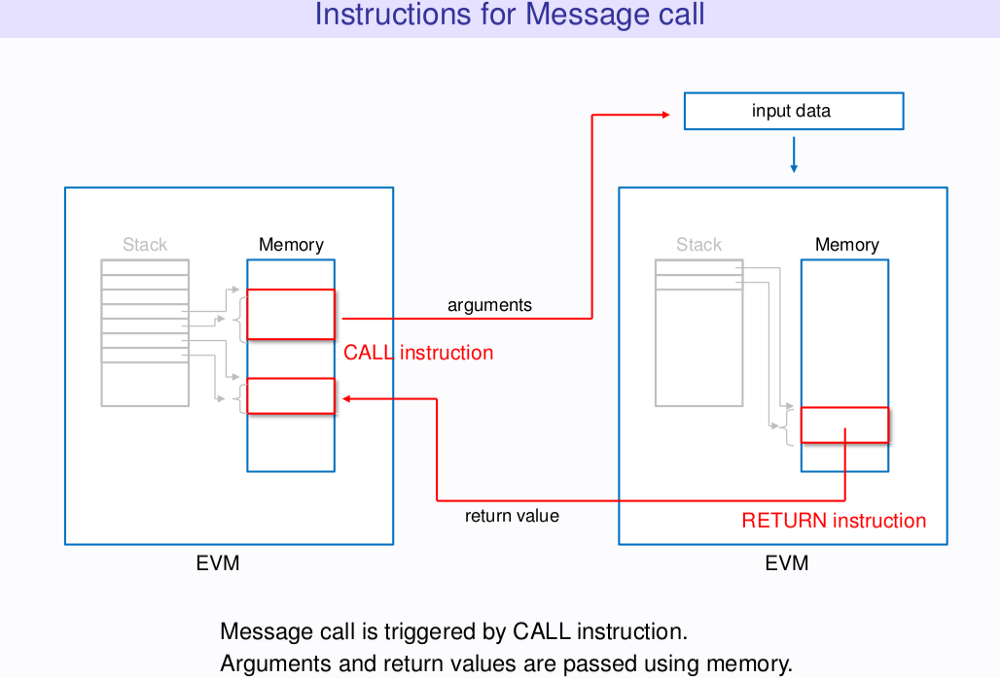Solidity为地址类型提供了原生调用方法，工作如下：

``````address.call.gas(gas).value(value)(data)
``````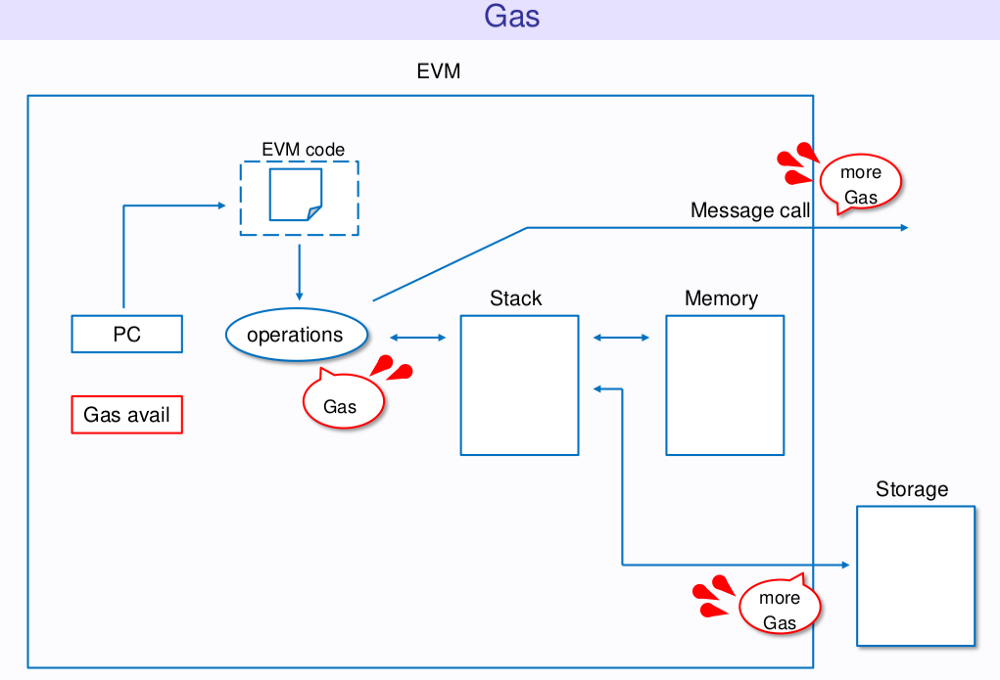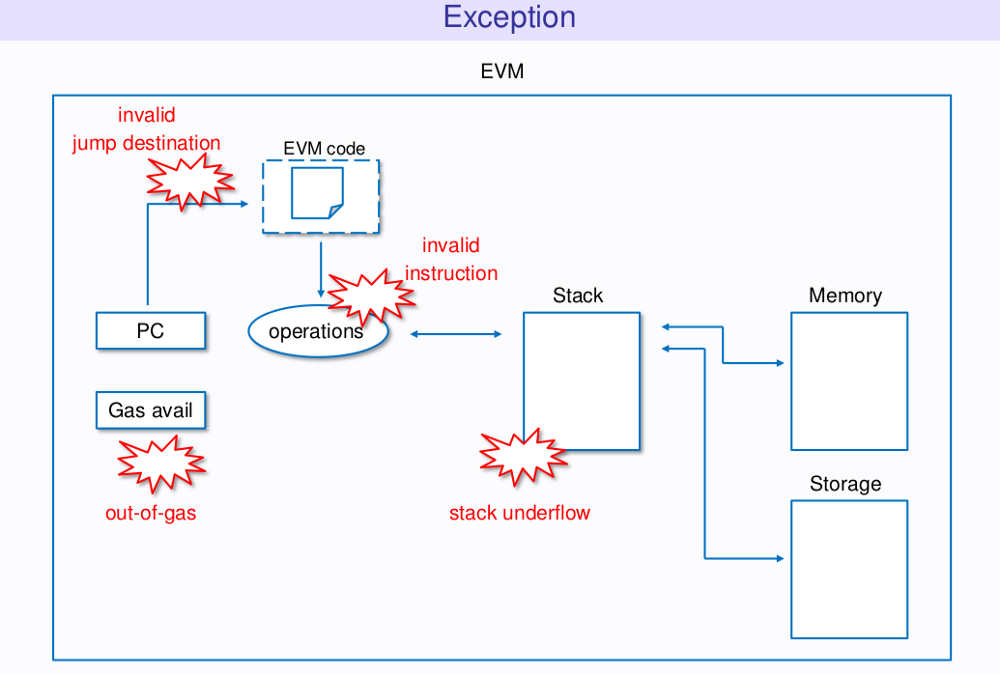``````contract Implementation {
event ImplementationLog(uint256 gas);
function() public payable {
emit ImplementationLog(gasleft());
assert(false);
}
}
contract Caller {
event CallerLog(uint256 gas);
Implementation public implementation;

function Caller() public {
implementation = new Implementation();
}
function () public payable {
emit CallerLog(gasleft());
implementation.call.gas(gasleft()).value(msg.value)(msg.data);
emit CallerLog(gasleft());
}
}
``````

``````truffle(develop)> compile
truffle(develop)> Caller.new().then(i => caller = i)
truffle(develop)> opts = { gas: 4600000 }
truffle(develop)> caller.sendTransaction(opts).then(r => result = r)
truffle(develop)> logs = result.receipt.logs
truffle(develop)> parseInt(logs.data) //4578955
truffle(develop)> parseInt(logs.data) //71495
``````

Solidity还提供了`call`操作码，支持在内联汇编（inline assembly）中管理调用：

``````call(g, a, v, in, insize, out, outsize)
``````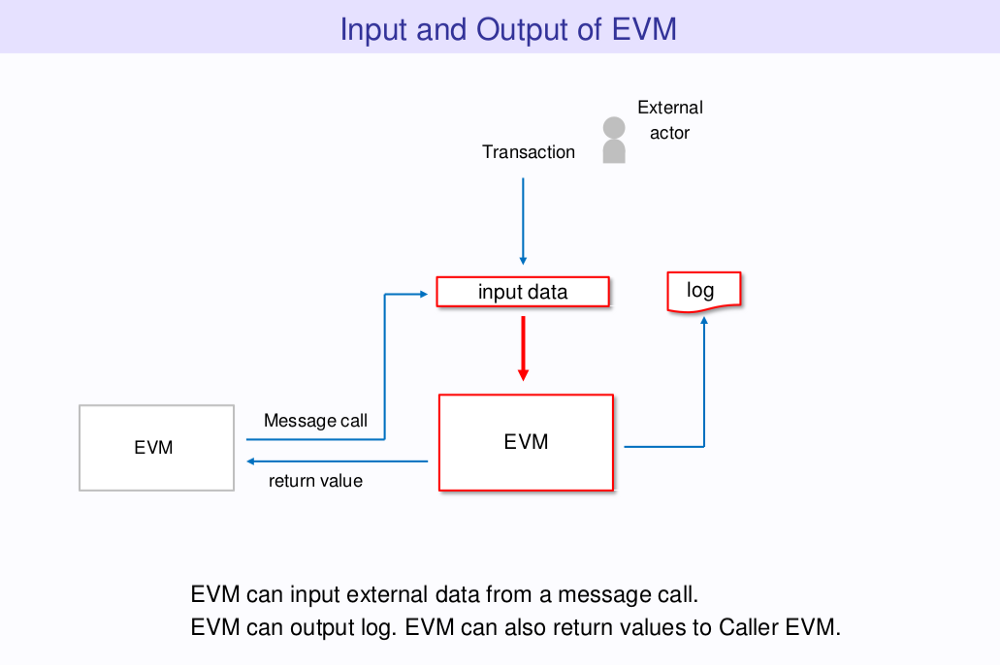EVM支持一类特殊的消息调用变体，称为“`delegatecall`”。同上，Solidity在提供内联汇编版本的同时，还提供了内建的地址方法。二者的不同之处在于，对于低层调用，目标代码在调用合约的上下文内执行，而`msg.sender``msg.value`并非如此。⁴

``````contract Greeter {
function thanks() public payable {
emit Thanks(msg.sender, msg.value);
}
}
``````

``````truffle(develop)> compile
truffle(develop)> someone = web3.eth.accounts
truffle(develop)> ETH_2 = new web3.BigNumber(‘2e18’)
truffle(develop)> Greeter.new().then(i => greeter = i)
truffle(develop)> opts = { from: someone, value: ETH_2 }
truffle(develop)> greeter.thanks(opts).then(tx => log = tx.logs)
truffle(develop)> log.event                     //Thanks
truffle(develop)> log.args.sender === someone   //true
truffle(develop)> log.args.value.eq(ETH_2)      //true
``````

``````contract Wallet {
Greeter internal greeter;

function Wallet() public {
greeter = new Greeter();
}

function () public payable {
bytes4 methodId = Greeter(0).thanks.selector;
require(greeter.delegatecall(methodId));
}
}
``````

``````truffle(develop)> Wallet.new().then(i => wallet = i)
truffle(develop)> wallet.sendTransaction(opts).then(r => tx = r)
truffle(develop)> logs = tx.receipt.logs
truffle(develop)> SolidityEvent = require(‘web3/lib/web3/event.js’)
truffle(develop)> Thanks = Object.values(Greeter.events)
truffle(develop)> event = new SolidityEvent(null, Thanks, 0)
truffle(develop)> log = event.decode(logs)
truffle(develop)> log.event                    // Thanks
truffle(develop)> log.args.sender === someone  // true
truffle(develop)> log.args.value.eq(ETH_2)     // true
``````

``````contract ResultStorage {
uint256 public result;
}
contract Calculator is ResultStorage {
Product internal product;
function Calculator() public {
product = new Product();
}

}
function mul(uint256 x) public {
bytes4 methodId = Product(0).calculate.selector;
require(product.delegatecall(methodId, x));
}
}
function calculate(uint256 x) public returns (uint256) {
uint256 temp = result + x;
assert(temp >= result);
result = temp;
return result;
}
}
contract Product is ResultStorage {
function calculate(uint256 x) public returns (uint256) {
if (x == 0) result = 0;
else {
uint256 temp = result * x;
assert(temp / result == x);
result = temp;
}
return result;
}
}
``````

``````truffle(develop)> Calculator.new().then(i => calculator = i)
truffle(develop)> calculator.product().then(a => productAddress = a)
truffle(develop)> calculator.result().then(r => r.toString()) // 5
truffle(develop)> addition.result().then(r => r.toString())   // 0
truffle(develop)> product.result().then(r => r.toString())    // 0
truffle(develop)> calculator.mul(2)
truffle(develop)> calculator.result().then(r => r.toString()) // 10
truffle(develop)> addition.result().then(r => r.toString())   // 0
truffle(develop)> product.result().then(r => r.toString())    // 0
``````

``````contract Implementation {
event ImplementationLog(uint256 gas);
function() public payable {
emit ImplementationLog(gasleft());
assert(false);
}
}
contract Delegator {
event DelegatorLog(uint256 gas);
Implementation public implementation;
function Delegator() public {
implementation = new Implementation();
}
function () public payable {
emit DelegatorLog(gasleft());
assembly {
calldatacopy(ptr, 0, calldatasize)
let result := delegatecall(gas, _impl, ptr, calldatasize, 0, 0)
}

emit DelegatorLog(gasleft());
}
}
``````

HackPedia: 16 Solidity Hacks/Vulnerabilities, their Fixes and Real World Examples

数据管理（Data Management）

EVM根据不同的应用场景，采用不同的方式管理不同类型的数据。除了合约代码之外，合约所管理的数据大体可分为四类：栈（Stack)、调用数据（calldata）、内存和存储。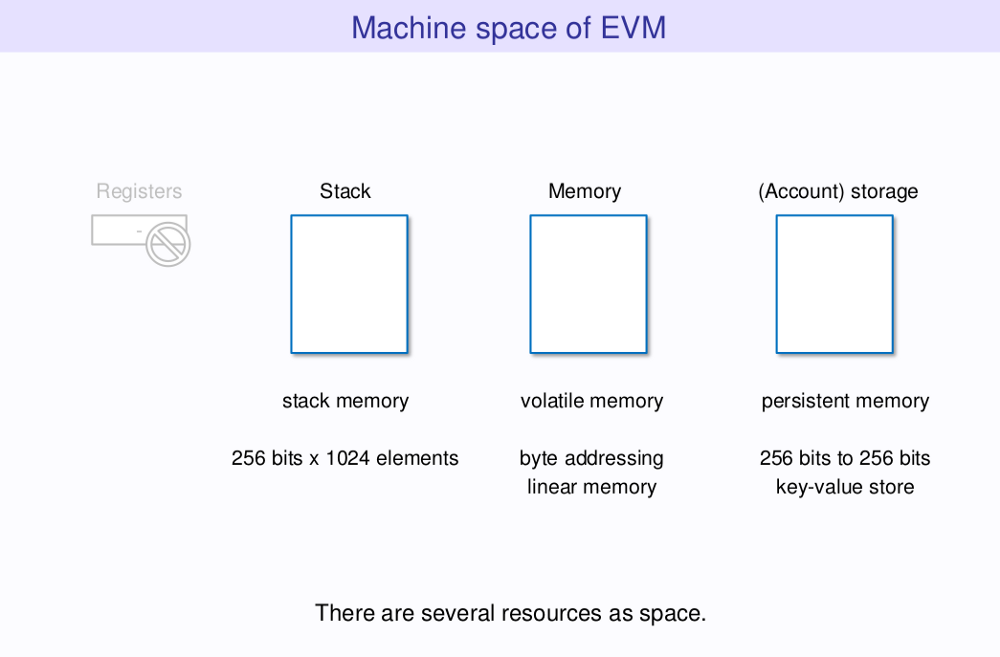栈（tack）

EVM本身就是一种栈机器。也就是说，EVM的操作并非基于注册函数，而是基于虚拟栈。栈的最大规模是1024，其中栈条目（item）的大小是256比特。事实上，EVM是一种256比特的字（word）机器，这种设计便于Keccak256哈希模式和椭圆曲线密码（elliptic-curve）的计算。下图给出了大部分操作码输入参数的来源。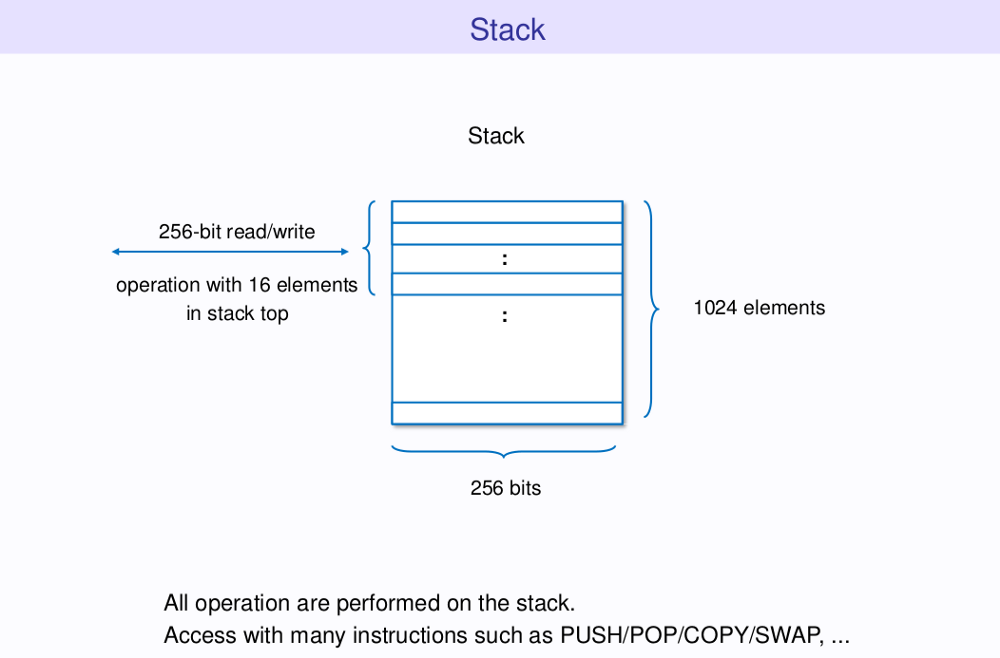EVM提供了多种操作码，用于直接操作栈。其中包括：

• POP：从栈中移除条目。
• PUSHn：将后面n个字节条目置于栈中，n的大小介于1到32之间。
• DUPn：复制第n个栈条目，n的大小介于1到32之间。
• SWAPn：交换栈中第一个和第n个条目的位置，n的大小介于1到32之间。

调用数据（Calldata）

• CALLDATASIZE：指定交易数据的规模。
• CALLDATACOPY：拷贝交易数据的指定字节数到内存中。

Solidity为上述操作码提供了内联编译版本，分别是`calldatasize``calldataload``calldatacopy`。其中，`calldatacopy`需要指定三个参数`(t, f, s)`，将`f`地址处的调用数据拷贝`s`个字节到`t`地址。此外，Solidity支持通过`msg.data`访问调用数据。

``````assembly {
calldatacopy(ptr, 0, calldatasize)
let result := delegatecall(gas, _impl, ptr, calldatasize, 0, 0)
}
``````

``````contract Calldata {
function add(uint256 _a, uint256 _b) public view
returns (uint256 result)
{
assembly {
calldatacopy(a, 4, 32)
}
}
}
``````

``````truffle(develop)> compile
truffle(develop)> Calldata.new().then(i => calldata = i)
truffle(develop)> calldata.add(1, 6).then(r => r.toString())    // 7
``````

内存（Memory）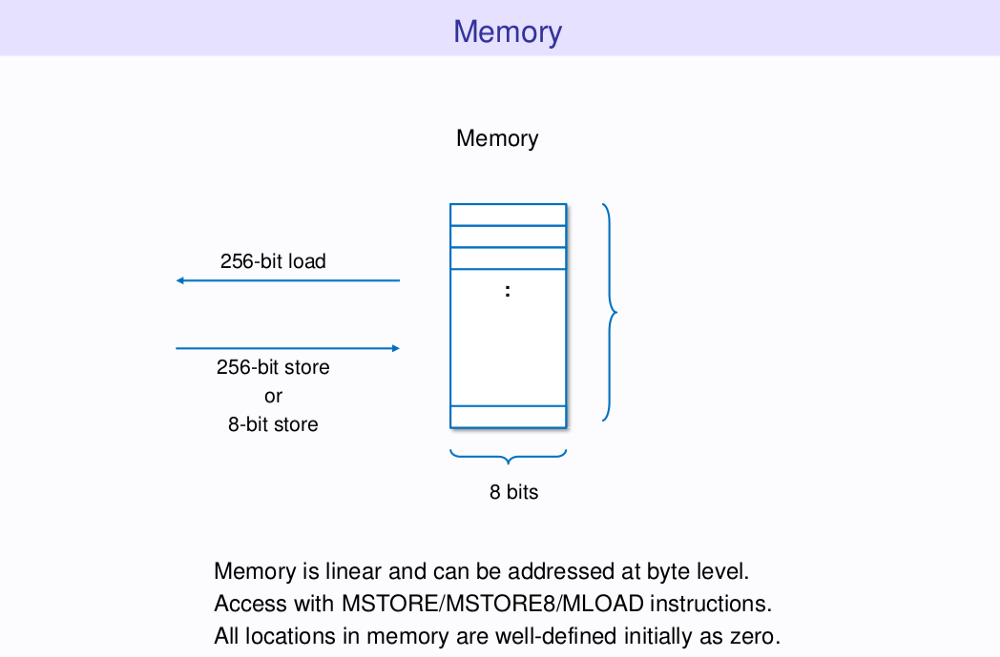EVM提供了三个操作内存区域的操作码：

• MSTORE：将字保存到内存中。
• MSTORE8：将一个字节保存到内存中。

Solidity同样对这些操作码提供了相应的内联汇编版本。

``````PUSH1   :  EVM操作码0x60
0x60    :  自由内存指针。
PUSH1   :  EVM操作码0x60。
0x40    :  自由内存指针的内存位置。
MSTORE  :  EVM操作码0x52。
``````

存储（Storage）

EVM提供了两个操作存储的操作：

• SSTORE：将字保存到存储。

Solidity自动将合约中每个已定义的状态变量映射到存储的相应插槽中。映射策略非常简单：固定大小的变量（即除映射和动态数组之外的所有变量）从存储的位置0开始连续布局。

``````contract Storage {
uint256 public number;
uint256[] private array;
mapping(uint256 => uint256) private map;
function Storage() public {
number = 2;
account = this;
array.push(10);
array.push(100);
map = 9;
map = 10;
}
}
``````

``````truffle(develop)> compile
truffle(develop)> Storage.new().then(i => storage = i)
``````

``````truffle(develop)> web3.eth.getStorageAt(storage.address, 0)  // 0x02
``````

``````truffle(develop)> web3.eth.getStorageAt(storage.address, 2)  // 0x02
``````

``````truffle(develop)> web3.eth.getStorageAt(storage.address, 3)
// 0x00
truffle(develop)> mapIndex = ‘0000000000000000000000000000000000000000000000000000000000000003’
truffle(develop)> firstKey = ‘0000000000000000000000000000000000000000000000000000000000000001’
truffle(develop)> firstPosition = web3.sha3(firstKey + mapIndex, { encoding: ‘hex’ })
// 0x09
truffle(develop)> secondKey = ‘0000000000000000000000000000000000000000000000000000000000000002’
truffle(develop)> secondPosition = web3.sha3(secondKey + mapIndex, { encoding: ‘hex’ })
// 0x0A
``````¹ 以太坊黄皮书中提出，“新账户地址定义为：对仅包含发送者和帐户nounce的结构做RLP编码，对所得到编码求Keccak哈希值，取该哈希值最右边开始的160位。”

² zeppelin_os的支柱之一就是合同的可升级性。 Zeppelin一直在探索实施这一目标的不同策略。 在这里阅读更多相关信息。

³ Solidity在调外部函数前，会先验证该函数的地址中是否具有字节码。否则，终止函数执行并还原状态。

作者简介Vaibhav Saini是一家由MIT Cambridge 创新中心孵化的初创企业TowardsBlockchain的联合创始人。Saini也是一名高级区块链开发人员，具有Ethereum、Quorum、EOS、Nano、Hashgraph、IOTA等多种区块链平台的开发经验。他目前是德里印度理工学院（IIT Delhi）的一名大二学生。

《深入EVM：EVM工作机制的深层分析（终极版）》上有2条评论

1.Horizon说道：

sandbox 国内翻译应该是沙盒、沙盘居多。沙箱略少一些吧？

1.Gai Lei说道：

OK,谢谢指出。已经修改译文。